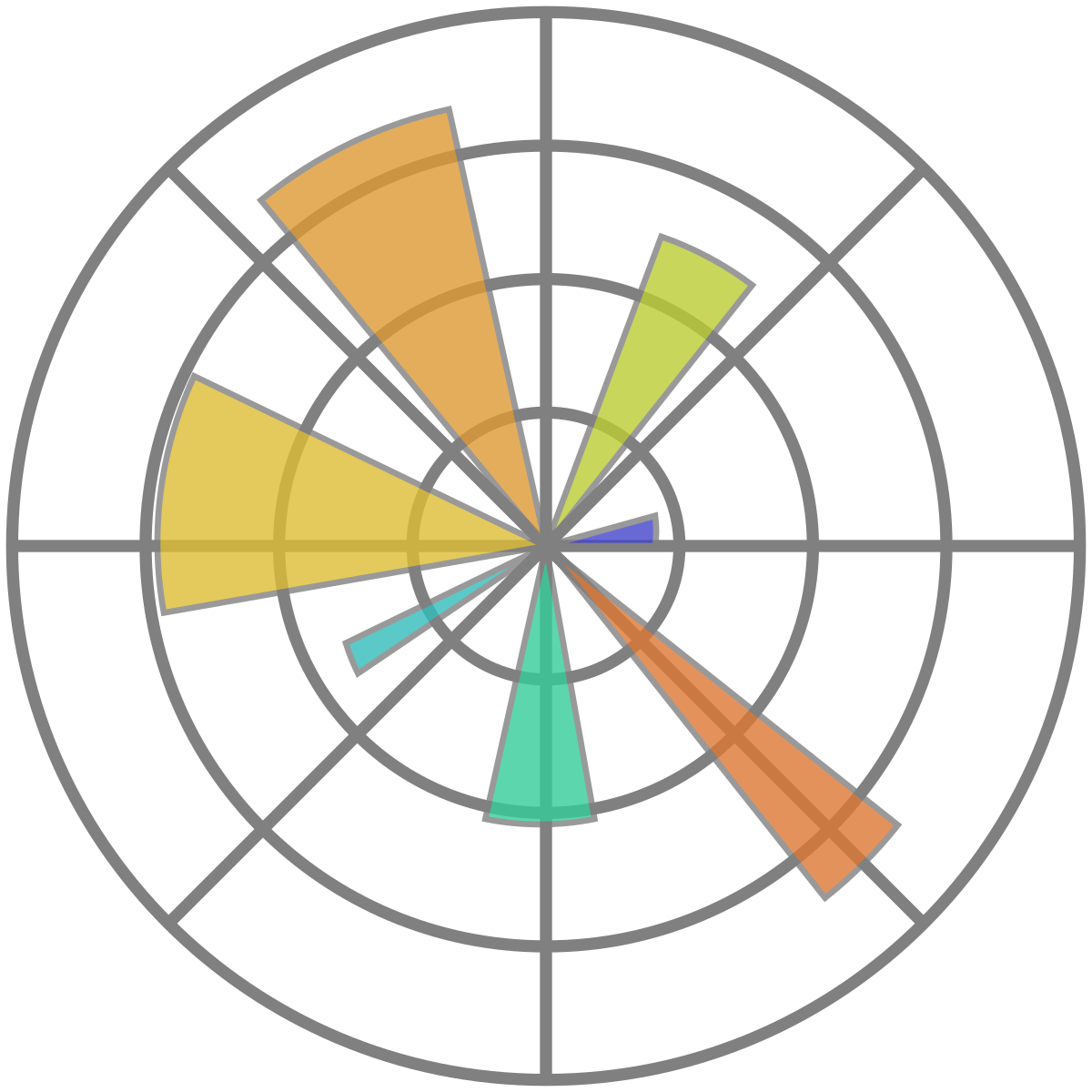# How do I edit an already existing diagram in matplotlib (python)?How do I edit an already existing diagram in matplotlib

To edit an already existing diagram in matplotlib, you can follow these steps:

1. Import the necessary modules:
`python`import matplotlib.pyplot as plt``

1. Retrieve the existing plot object:

If you have the `Figure` object that the plot was created on, you can retrieve the plot object using the `axes` attribute of the `Figure` object. For example:

`python`fig, ax = plt.subplots() ``# ... create the plot ...``

1. In this case, the `ax` variable is the plot object that you can modify.

If you don't have the `Figure` object, but you know the name of the plot that you want to modify, you can retrieve the plot object using the `plt.gca()` function, which gets the current axes (plot) instance. For example:

```python```plt.plot(x, y, label='my plot')
ax = plt.gca()  # retrieve the plot object``````

In this case, the `ax` variable is the plot object that you can modify.

3. Modify the plot:

Once you have the plot object, you can modify it using its methods. For example, to change the label of a line plot, you can use the `set_label()` method:

```python```ax.lines.set_label('new label')
``````

Here, `ax.lines` refers to the first line in the plot, and `set_label()` sets its label to 'new label'.

To change the title of the plot, you can use the `set_title()` method:

```python```ax.set_title('new title')
``````

To change the x-axis label, you can use the `set_xlabel()` method:

```python```ax.set_xlabel('new x-axis label')
``````

And so on. There are many other methods that you can use to modify the plot object, depending on what you want to change.

1. Update the plot:

After modifying the plot object, you need to update the plot to see the changes. If you're using an interactive backend (e.g. `plt.ion()`), the plot will update automatically. If not, you can use the `plt.draw()` or `plt.show()` functions to update the plot.

For example:

`python`plt.draw()  # update the plot``

or:

`python`plt.show()  # update the plot and display it``

That's it! You should now be able to edit an already existing diagram in matplotlib.

Conclusion

Matplotlib is a powerful library for creating and modifying plots in Python. With the ability to retrieve plot objects and use their methods, modifying existing diagrams is a straightforward process. This can be useful for making updates to existing visualizations or customizing plots to better suit specific needs.